## Sunday, July 17, 2011

### Bugs, roots, words

I'm going to wait until next weekend to post the second half of that last entry. I've had too much else to juggle this past week to produce anything more than 75% of a rough draft, and I'd rather not just rush it out without thinking it over a bit more.

In the meantime, I do have some other stuff for you. First, an update to that earlier piece about those neat little buggers called damselflies.

I had previously thought male ebony jewelwings came in two colors: blue and green. Turns out that they actually come in one color: blue and green.

Here is a photo of a male I snapped earlier today:And this is a shot of the same bug, taken about two minutes later:My hunch was correct: whether they appear green or blue depends on the light bouncing off of them. From what I've observed, they're blue when they're closer to water, and green when they're around leafy plants. Whether this is caused by the different amounts of sunlight hitting them (being near the water tends to put them out of the shade, after all) or by the general color of their surroundings, I'm not sure -- but I'm interested in looking further into it. (I suspect the first guess is probably the more accurate.)

Again: this all probably helps explain why I don't have a girlfriend.

Anyway. While visiting Manhattan last night to help a couple of friends lug their amps and synthesizers, I happened to pass a table of books on the sidewalk being sold for a buck each. The broad span of the collection consisted of dry periodicals, hardcover books about statistics, issues of comic books published by Valiant, and lousy fiction paperbacks about love and murder. Only one tome caught my eye: Constance Reid's A Long Way from Euclid, a 1963 hardcover about classical geometry's long and continuous influence upon the development of mathematics.

I spent a lot of time flipping through it between bands and on the ride back home. Just about everything in the later chapters goes over my head, but the first few sections -- which are much, much more elementary and therefore less terrifying to a reader who consistently got Cs and Ds in every math class from algebra I to precalculus -- were great fun to read.

If you read last week's entry, you'll remember my kvetching and complaining about how students are taught the discoveries of science without learning the methods or much of the history. The same is true with mathematics -- maybe even more so, if what I recall from my own primary school years suggests a wider trend.

I, like most students, was shown a right triangle in geometry class, told that a2 + b2 = c2 , and then commenced solving twenty worksheets of right triangle problems. What we didn't learn was the history of the Pythagorean theorem and its tremendous influence upon the course of Western mathematics and thought -- topics any pupil would benefit from knowing. (I learned about these details a few years later during a "history of mathematics" course I took in college as a means to fulfill a math requirement without giving my abysmal computative abilities the chance to destroy my GPA.)

A Long Way from Euclid's first chapter discusses some of the Pythagorean theorem's immediate effects, and I've prepared an excerpt for your reading pleasure. You will either find it really interesting or boring as hell. There is not much likely middle ground.

Thus, five hundred years before the birth of Christ, mathematics had in hand its famous theorem about the square on the hypotenuse of the right triangle -- a theorem which was destined, in the words of E. T. Bell, to run "like a golden thread" through all of its history. This theorem would serve -- in trigonometry, which is entirely based on it -- as the tool for measurement lying beyond the immediate use of tape measure and ruler. In analytic geometry, it would serve as the basic distance formula for space in any number of dimensions. In its arithmetical generalization (an + bn = cn), it would provide mathematics with is most famous unsolved problem, known as Fermat's Last Theorem. In the most revolutionary mathematical discovery of the nineteenth century, it would be revealed as the equivalent of the distinguishing axiom of Euclidean geometry; and in our own century it would be further generalized so as to be appropriate to and include geometries other than that of Euclid. Twenty-five hundred years after its first general statement and proof, the theorem of Pythagoras would be found, firmly embedded, in Einstein's theory of relativity.

But we are getting ahead of our story. For the moment we are concerned only with the fact that the discovery and proof of the Pythagorean theorem was directly responsible for setting the general direction of Western mathematics.

We have seen how the Pythagoreans lived and discovered their great theorem under the unchallenged assumption that Number Rules the Universe. When they said Number, they meant whole number: 1, 2, 3. . . . Although they were familiar with the sub-units which we call fractions, they did not consider these numbers as such. They managed to transform them into whole numbers by considering them, not as parts, but as ratios between two whole numbers. (This mental gymnastic has led to name rational numbers for fractions and integers, which are fractions with a denominator equal to one.) Fractions disposed of as ratios, all was right with the world and Number (whole number) continued to rule the Universe. The gods were mathematicians -- arithmeticians. But, all the time unsuspected, there was numerical anarchy afoot. That it should reveal itself to the Pythagoreans through their own most famous theorem is one of the great ironies in mathematical history. The golden thread began in a knot.

The Pythagoreans had proved by the laws of logic that the square on the hypotenuse of the right triangle is equal to the sum of the squares on the other two sides. They had also discovered the general method by which they could obtain solutions in whole numbers for all three side of such a triangle. Although these whole number triples (the smallest being 3, 4, 5) still bear the name of "the Pythagorean numbers," the Pythagoreans themselves knew that not all right triangles had whole-number sides. They assumed, however, that the sides and hypotenuse of any right triangle could always be measured in units and sub-units which could then be expressed as the ratio of whole numbers. For, after all, did not Number -- whole number -- rule the Universe?

Imagine then the Pythagoreans' dismay when one of their society, observing the simplest of right triangles, that which is formed by the diagonal of the unit square, came to the conclusion and proved it by the inexorable process of reason, that there could be no whole number or ratio of whole numbers for the length of the hypotenuse of such a triangle: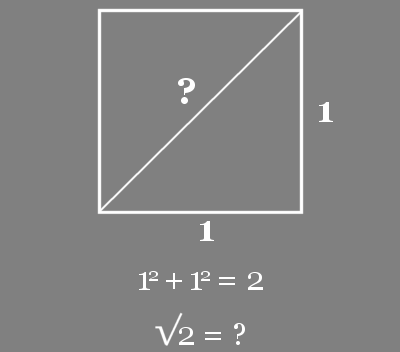When we look at any isosceles right triangle -- and remember that the size is unimportant, for the length of one of the equal sides can always be considered the unit of measure -- it is clear that the hypotenuse cannot be measured by a whole number. We know by the theorem of Pythagoras that the hypotenuse must be equal to the square root of the sum of the squares of the other two sides. Since 12 + 12 = 2, the hypotenuse must be equal to √2. Some number multiplied by itself must produce 2. What is this number?

It cannot be a whole number, since 1 x 1 = 1 and 2 x 2 = 4. It must then be a number between 1 and 2. The Pythagoreans had always assumed that it was a rational "number." When we consider that the rational numbers between 1 and 2 are so numerous that between any two of them we can always find an infinite number of other rational numbers, we cannot blame them for assuming unquestioningly that among such infinities upon infinities there must be some rational number which when multiplied by itself would produce 2. Some of them actually pursued √2 deep into the rational numbers, convinced that, somewhere among all those rational numbers, there must be one number -- one ratio, whole number to whole number -- which would satisfy the equation we would write today as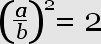The closest they came to such a number was 17/12, which when multiplied by itself produces 289/144, or, 2 1/144.

But one of the Pythagoreans, a man truly ahead of his time, stopped computing and considered instead another possibility. Perhaps there is no such number.

Merely considering such a possibility must be rated as an achievement. In some respects it was an even greater achievement than the discovery and proof of the famous theorem that produced the dilemma!

Perhaps there is no such number. How does a mathematician go about proving that there isn't a solution to the problem he is called upon to solve? The answer is classic. He simply assumes that what he believes to be false is in actuality true. He then proceeds to show that such an assumption leads to a contradiction, usually with itself, and of necessity cannot be true. This method has been vividly called proof per impossible or, more commonly, reductio ad absurdum. "It is," wrote a much more recent mathematician than the Pythagorean, "a far finer gambit than a chess gambit: a chess player may offer the sacrifice of a pawn or even a piece, but a mathematician offers the game."

The most recent proof to shake the foundations of mathematical thought was based on a reductio and so, twenty-five hundred years ago, was the first. We shall present this proof, which is a fittingly elegant one for so important an idea, in the notation of modern algebra, although this notation was not available to the man who first formulated the proof.

Let us assume that, although we know we have never been able to find it, there actually is a rational number a/b which when multiplied by itself produces 2. In other words, let us assume there exists an a/b such that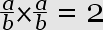We shall assume (and this is the key point in the proof) that a and b have no common divisors. This is a perfectly legitimate assumption, since if a and b had a common divisor we could always reduce a/b to lowest terms. Now, saying thatis the same as saying that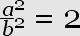If we multiply both sides of this equation by b2 (which we can, since b does not equal 0 and since we can do anything to an equation without changing its value as long as we do the same thing to both sides), we shall obtain: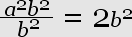or, by canceling out the common divisor b2 on the left-hand side: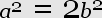It is obvious, since a2 is divisible by 2, that a2 must be a even number. Since odd numbers have odd squares, a must also be an even number. If a is even, there must be some other whole number c which when multiplied by 2 will produce a; for this is what we mean by a number being "even." In other words,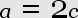If we substitute 2c for a in the equation a2 = 2b2, which we obtained above, we find that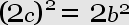or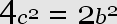Dividing both sides of this equation by 2, we obtain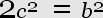Therefore, b2 like a2 in our earlier equation, must also be an even number; and it follows that b, like a, must be even.

BUT (and here is the impossibility, the absurdity which clinches the proof) we began by assuming that a/b was reduced to lowest terms. If a and b are both even, they must -- be definition of evenness -- have the common factor 2. Our assumption that there can be a rational number a/b which when multiplied by itself produces 2 must be false; for such an assumption leads us into a contradiction: we begin by assuming a rational number reduced to lowest terms and end by proving that the numerator and denominator are both divisible by 2!

We can only imagine with what consternation this result was received by the other Pythagoreans. Mysticism and mathematics were met on a battleground from which there could be no retreat and no compromise. If the Universe was indeed ruled by Number, there must be a rational number a/b equal to √2. But by impeccable mathematical proof one of their members had shown that there could be no such number!

The Pythagoreans had to recognize that the diagonal of so simple a figure as the unit square was incommensurable with the unit itself. It is no wonder they called √2 irrational! It was not a rational number, and it was contrary to all they had believed rational, or reasonable. The worst of the matter was that √2 was not by any means the only irrational number. They went on to prove individually that the square roots of 3, 5, 6, 7, 8, 10, 11, 12, 13, 14, 15 and 17 were also irrational. Although they worked out a very ingenious method of approximating such irrational values by way of ratios...they had to face the fact that there was not just one, there were many (in fact, infinitely many) lengths for which they could find no accurate numerical representation in a Universe that was supposedly ruled by Number.

Tradition tells us that they tried to solve their dilemma by persuading the discoverer of the unpleasant truth about √2 to drown himself. But the truth cannot be drowned so easily; nor would any true mathematician, unconfused by mysticism, wish to drown it. The Pythagoreans and the mathematicians who followed them, from Euclid to Einstein, had to live and work with the irrational.

Here was the golden thread impossibly knotted at its very beginning!

It was at this point that the Pythagoreans, rather than struggling to unravel arithmetically what must have seemed to them a veritable Gordian knot, took the way out that a great soldier was to take in a similar situation. They cut right through the knot. If they could not represent √2 exactly by a number, they could represent it exactly by a line segment. For the diagonal of the unit square is √2.

With the choice of two mathematical roads before them, the Greeks, long before the time of Euclid, chose the geometric one; and
"That has made all the difference."

I'd say the book was worth the buck.

#### 2 comments:

1.I was just reading about Pythagoras and having similar thoughts. I got terrible marks in math in high school, and yet, I think if any of my lessons had been linked to history in some way, I'd have gotten a better hold of it. I'm only discovering (slowly) an interest in math now. Eh, I could go on about the American education system, mais alas...

(I've been lurking your blog, by the way. I'm Marchelebration from the tweeter.)

2.Hi! I just think it's neat for how it implies that there's evidently irrationality hardwired into the universe...in a manner of speaking.

I've been revisiting math because of this whole "I want to learn about the stars" business. The deeper into it I get, the more necessary it is that I have some understanding of advanced mathematics.

I guess the stuff that's most worth doing is rarely the easiest, right?